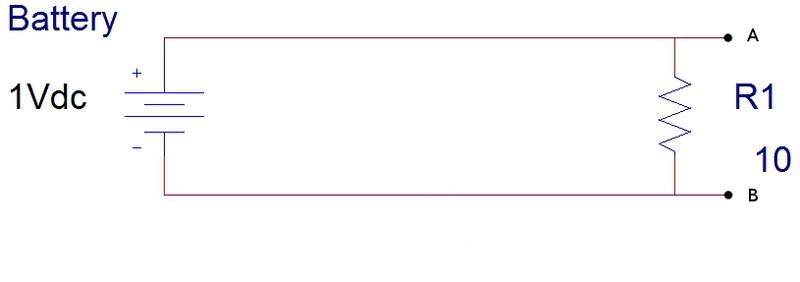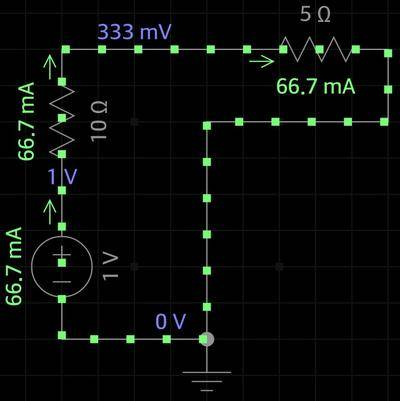# Why is my circuit reducing the voltage to 0?

• Psinter

#### Psinter

I have what may be a simple question for some, but for me... I just can't figure it out. I have a AA battery which my multimeter is reading to have 1.0V. When I use the battery as shown in the image below (in parallel with a 10Ω resistor) my voltage through the resistor (measured at points A and B) shows to be 0V.

However, when I connect a bigger resistor (10kΩ), the voltage across the resistor shows 0.68V. The voltage keeps increasing if I keep increasing the resistor value.

Why is my voltage being reduced to 0 when the resistor in parallel is of low value, but increases when the resistor has a bigger value?

The circuit:Edit: I fixed a typo there.

Last edited:

What type of battery is it? Is it a recharageble battery?

What type of battery is it? Is it a recharageble battery?
Yes, it is a rechargeable battery.

Yes, it is a rechargeable battery.
to have 1.0V
It is a dead rechargeable battery.

It is a dead rechargeable battery.
Ok, but still, I don't get why it shows 0V when connected to a 10Ω, but 0.68V when connected to a 10kΩ resistor. In this circuit: The higher the resistance, the higher the voltage; I don't get why.

EDIT: The higher is the voltage at terminals A and B. I forgot to specify that in my last sentence.

Multimeters have a very high internal impedance and put very little load on the battery, so even a dead battery can read more than 0. If a 1.5V battery is reading 1.0V, you KNOW it's pretty much totally discharged. Your load test isn't even necessary.

AA is nominally 1.5 v. The internal resistance of the battery is enough larger than ten ohms that there is no measurable voltage drop across the resistor. As you increase the resistance, the proportion of the 1.5 V that is dropped across the resistor increases.

If you charge a rechargeable battery for a few minutes, you get a "surface charge" on the battery. If you connect the battery to your circuit using a low resistance, the charge quickly drains off and by the the time you read your meter, the charge is nearly zero. When you use a large resistance, the charge doesn't drain quickly and so you get a higher reading.

•Psinter
Real voltage sources have an internal resistance in series with the ideal voltage source. By measuring the output voltage at several output currents, you can calculate the internal resistance. When the battery gets discharged, the internal resistance goes up, so you get lower and lower output voltages into low values of load resistance. Make sense?

Are we all that bored with the game?

Which what game?

If you charge a rechargeable battery for a few minutes, you get a "surface charge" on the battery. If you connect the battery to your circuit using a low resistance, the charge quickly drains off and by the the time you read your meter, the charge is nearly zero. When you use a large resistance, the charge doesn't drain quickly and so you get a higher reading.
Oh. Now I get it. Thank you very much.
Real voltage sources have an internal resistance in series with the ideal voltage source. By measuring the output voltage at several output currents, you can calculate the internal resistance. When the battery gets discharged, the internal resistance goes up, so you get lower and lower output voltages into low values of load resistance. Make sense?
Not really. I understood Stephen post better. Let me read it and re-read it a couple times and see if I get it. I'm actually a little slow.

Psinter,

My post is relevant if you are charging the battery for a few minutes and then doing the test. If you are not charging the battery, Bystander's explanation is better. Draw a circuit diagram of what Bystander described (put another resistor in series with a 1V battery, so the circuit has 2 resistors in it.)

Psinter,

My post is relevant if you are charging the battery for a few minutes and then doing the test. If you are not charging the battery, Bystander's explanation is better. Draw a circuit diagram of what Bystander described (put another resistor in series with a 1V battery, so the circuit has 2 resistors in it.)

Oh, yes yes yes. The measurements match now when I analyze the circuit. I get millivolts in the analysis, but in practice my multimeter doesn't show millivolts so instead I see 0V.

When the 2nd resistor is lower than the first one (connected in series) I get a very low voltage on it.

Thanks guys. This is solved then. To resume for anyone who might come with the same problem, here is the situation in my own words as I understood:

The voltage source is not supplying enough current. Because the voltage source is a battery, it has an internal resistance and if you re-draw my first circuit (shown in the first post), but this time taking in consideration the internal resistance of the battery. You get a circuit like in the image below:Here I drew the internal resistance of the battery to be 10Ω and the external to be 5Ω. Different from my original problem, but equivalent for explanation purposes.

We know that V = IR. Calculate the voltage for each resistor using Ohm's Law and you will see that the external resistance (5Ω) has a lower voltage than the internal resistance. If you change the 5Ω resistor to be bigger than 10Ω in this problem, you will get a bigger voltage because of Ohm's Law (notice that current stays constant).

Given that my battery is discharged, the current flow is extremely low, that's why the voltage reduces to 0V. (Actually a few millivolts according to Ohm's Law, but my multimeter lacks precision and I only see 0V).

To resume the resume: low current supplied by a battery through a resistor load will render a very low voltage on the load, given that the resistor load is lower than the internal resistance of the battery since the circuit is basically a voltage divider. Increase the resistor load to be more than the internal resistance of the battery and the voltage will obviously be higher.

Last edited:
If you change the 5Ω resistor to be bigger than 10Ω in this problem, you will get a bigger voltage because of Ohm's Law (notice that current stays constant).

No, if you change the external resistor to from 5 Ohms to 10 Ohms, resistance is doubled therefore current through it is halved

generally the battery's internal resistance is not taken into account as it isn't known ( tho it could be calculated / estimated by what maximum current the battery can supply for its voltage rating).
rather we just say that the battery has a voltage of 1.5V (or whatever) and there will be xx current through a given load
The voltage drop across the load will be 1.5V ( that will decrease in time as the battery goes flat)

Dave

Last edited:
Batteries are not like charged capacitors.Hence the explanation cannot be based on drain of charges when we connect a small resistance across it. Batteries or any other voltage source generates voltage through charge separation, i.e pushing positive charges to one terminal and negative to other.Dynamos do it through magnetic lorenz force experienced in a conductor moving in magnetic field.For batteries it is through chemical forces.And they have to spend some energy for doing so

When current flows in the circuit, the flowing charges tend to neutralise this charge separation ,as the positive charges flow from positive terminal to negative terminal through the external circuit.To maintain the same potential difference , the battery has to spend some energy in separating the charges again.If battery is weak, it will not be able to do this work if there is a large current .(rate of energy spent(power) =Charge per second X voltage to be maintained).If the battery is open circuited, only a momentary separation of charges is needed and no continuous energy to be spend.Just like a loaded spring.Hence, even if battery shows 1V during open circuit, it is unable to maintain it when a large current flows

Last edited: